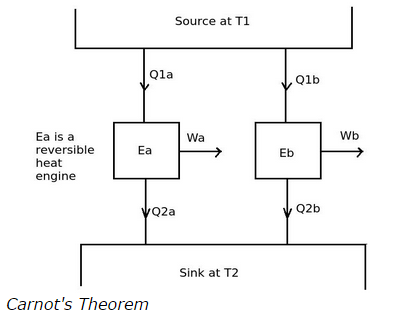# As per Carnot's theorem, efficiency of a reversible heat engine operating between a same given constant temperature

## According to the Carnot's theorem, the efficiency of a reversible heat engine operating between a same given constant temperature source and a given constant temperature sink is

Options:

Correct Answer: a. higher than any other irreversible heat engine

a. higher than any other irreversible heat engine
b. less than any other irreversible heat engine
c. equal to any other irreversible heat engine
d. none of the above

Explanation

The Carnot's theorem states that no other heat engine has a higher efficiency that a reversible engine, when all the heat engines are operated between a given constant temperature source and a given constant temperature sink.Consider the above diagram, the heat engines Ea and Eb are operating between the same constant temperature heat source and constant temperature sink. If heat engine Ea is a reverse heat engine and Eb is any other heat engine then according to the Carnot's theorem the efficiency of Ea is always greater than the efficiency of Eb.